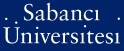# Application of a general risk management model to portfolio problems with elliptical distributions /

Kaynar, Bahar (2006) Application of a general risk management model to portfolio problems with elliptical distributions /. [Thesis]Preview
PDF - Requires a PDF viewer such as GSview, Xpdf or Adobe Acrobat Reader
428Kb

## Abstract

Focus is directed to a class of risk measures for portfolio optimization with two types of disutility functions, where the random return variables of financial instru¬ments are assumed to be distributed by multivariate elliptical distributions. Recent risk measures, Value-at-Risk (VaR) and Conditional Value-at-Risk (CVaR) are consid¬ered under this setting. If the joint distribution function of the financial instruments is elliptical and disutility is taken as linear reflecting the behavior of risk neutral in¬vestors, then the optimal solution of the mathematical models with objective functions formed by VaR and CVaR measures, is equivalent to the solution of the corresponding Markowitz model. To solve the Markowitz model, a very fast, finite step algorithm proposed in the literature, has been modified and implemented . Finally, the CVaR model with convex increasing disutility functions reflecting the behavior of risk averse investors has been introduced. Although, a convex objective function exists in this case, an analytic form cannot be obtained. However, unlike generating scenarios from multivariate distributions as suggested in the literature, the objective function can be closely estimated by simulating realizations only from univariate distributions. The thesis concludes with a thorough computational study on a sample data collected from the Istanbul Stock Exchange for each different class introduced.

Item Type: Thesis Elliptical distributions -- Risk management -- Linear loss functions -- Value-at-risk -- Conditional value-at-risk -- Portfolio optimization -- Disutility --Elliptical T Technology > T Technology (General) 8325 IC-Cataloging 15 Apr 2008 16:59 25 Mar 2019 16:54

Repository Staff Only: item control page